### Home > CALC > Chapter 4 > Lesson 4.3.2 > Problem4-113

4-113.
1. A horizontal flag is shown below. Homework Help ✎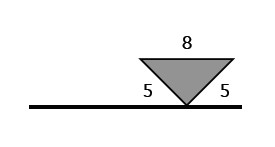1. Imagine rotating the flag about its pole and describe the resulting three-dimensional figure. Draw a picture of this figure on your paper.

2. Find the volume of the rotated flag.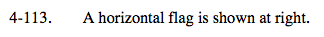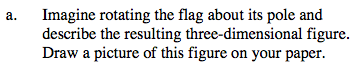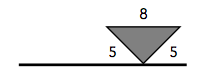Imagine a cylinder with cones carved out of the top and bottom.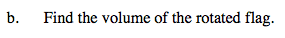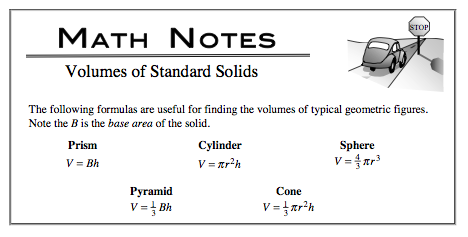$\text{Volume = cylinder }-2(\text{cone})=\pi r^{2}h-2\left ( \frac{1}{3}\pi r^{2}h \right )$

$48\pi\text{ units}^{3}$

Use the eTool below to visualize the flag.
Click the link at right for the full version of the eTool: Calc 4-113 HW eTool